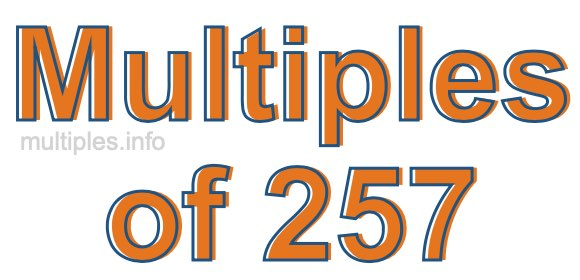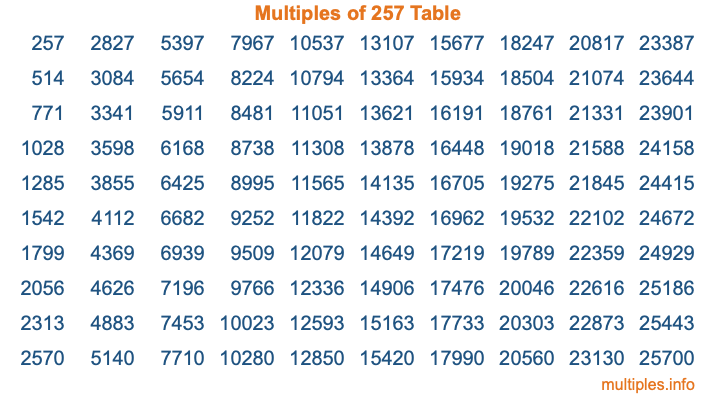Multiples of 257Welcome to the Multiples of 257 page. Here we will first teach you everything you will ever need to know about the multiples of 257, and then give you a study guide summary of everything we taught you to make sure you remember it all. Use this page to look up facts and learn information about the multiples of 257. This page will make you a multiples of two hundred fifty-seven expert!

Definition of Multiples of 257
Multiples of 257 are all the numbers that when divided by 257 equal an integer. Each of the multiples of 257 are called a multiple. A multiple of 257 is created by multiplying 257 by an integer.

Therefore, to create a list of multiples of 257, you start with 1 multiplied by 257, then 2 multiplied by 257, then 3 multiplied by 257, and so on for as long as you want. Thus, the list of the first five multiples of 257 is 257, 514, 771, 1028, and 1285. To see a larger list of multiples of 257, see the printable image of Multiples of 257 further down on this page. We also have a category where you can choose any nth multiple of 257.

Multiples of 257 Checker
The Multiples of 257 Checker below checks to see if any number of your choice is a multiple of 257. In other words, it checks to see if there is any number (integer) that when multiplied by 257 will equal your number. To do that, we divide your number by 257. If the the quotient is an integer, then your number is a multiple of 257.

Is  a multiple of 257?

Least Common Multiple of 257 and ...
A Least Common Multiple (LCM) is the lowest multiple that two or more numbers have in common. This is also called the smallest common multiple or lowest common multiple and is useful to know when you are adding our subtracting fractions. Enter one or more numbers below (257 is already entered) to find the LCM.

Check out our LCM Calculator if you need more details about the Least Common Multiple or if you need the LCM for different numbers for adding and subtraction fractions.

nth Multiple of 257
As we stated above, 257 is the first multiple of 257, 514 is the second multiple of 257, 771 is the third multiple of 257, and so on. Enter a number below to find the nth multiple of 257.

th multiple of 257

Multiples of 257 vs Factors of 257
257 is a multiple of 257 and a factor of 257, but that is where the similarities end. All postive multiples of 257 are 257 or greater than 257. All positive factors of 257 are 257 or less than 257.

Below is the beginning list of multiples of 257 and the factors of 257 so you can compare:

Multiples of 257: 257, 514, 771, 1028, 1285, etc.

Factors of 257: 1, 257

As you can see, the multiples of 257 are all the numbers that you can divide by 257 to get a whole number. The factors of 257, on the other hand, are all the whole numbers that you can multiply by another whole number to get 257.

It's also interesting to note that if a number (x) is a factor of 257, then 257 will also be a multiple of that number (x).

Multiples of 257 vs Divisors of 257
The divisors of 257 are all the integers that 257 can be divided by evenly. Below is a list of the divisors of 257.

Divisors of 257: 1, 257

The interesting thing to note here is that if you take any multiple of 257 and divide it by a divisor of 257, you will see that the quotient is an integer.

Multiples of 257 Table
Below is an image of the first 100 multiples of 257 in a table. The table is in chronological order, column by column. The first column has the first ten multiples of 257, the second column has the next ten multiples of 257, and so on.The Multiples of 257 Table is also referred to as the 257 Times Table or Times Table of 257. You are welcome to print out our table for your studies.

Negative Multiples of 257
Although not often discussed or needed in math, it is worth mentioning that you can make a list of negative multiples of 257 by multiplying 257 by -1, then by -2, then by -3, and so on, to get the following list of negative multiples of 257:

-257, -514, -771, -1028, -1285, etc.

Multiples of 257 Summary
Below is a summary of important Multiples of 257 facts that we have discussed on this page. To retain the knowledge on this page, we recommend that you read through the summary and explain to yourself or a study partner why they hold true.

There are an infinite number of multiples of 257.

A multiple of 257 divided by 257 will equal a whole number.

257 divided by a factor of 257 equals a divisor of 257.

The nth multiple of 257 is n times 257.

The largest factor of 257 is equal to the first positive multiple of 257.

257 is a multiple of every factor of 257.

257 is a multiple of 257.

A multiple of 257 divided by a divisor of 257 equals an integer.

257 divided by a divisor of 257 equals a factor of 257.

Any integer times 257 will equal a multiple of 257.

Multiples of a Number
Here you can get the multiples of another number, all with the same attention to detail as we did for multiples of 257 on this page.

Multiples of
Multiples of 258
Did you find our page about multiples of two hundred fifty-seven educational? Do you want more knowledge? Check out the multiples of the next number on our list!